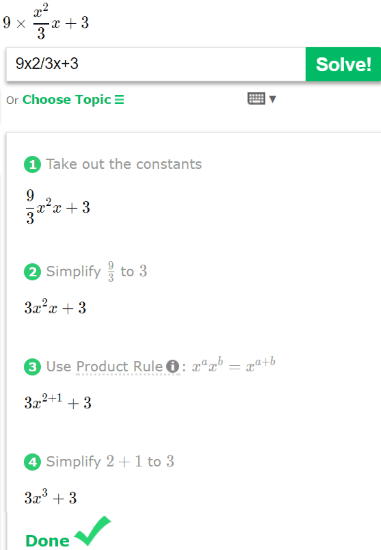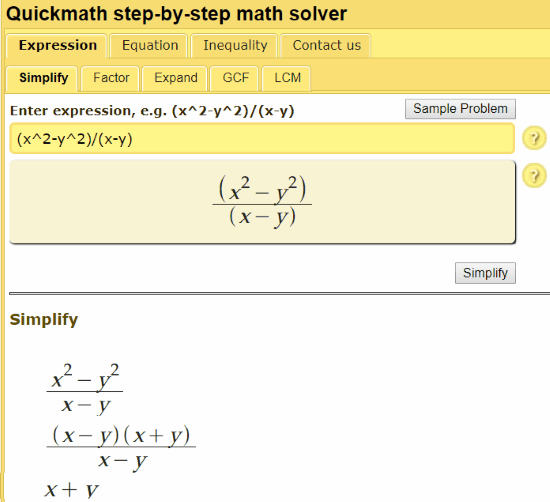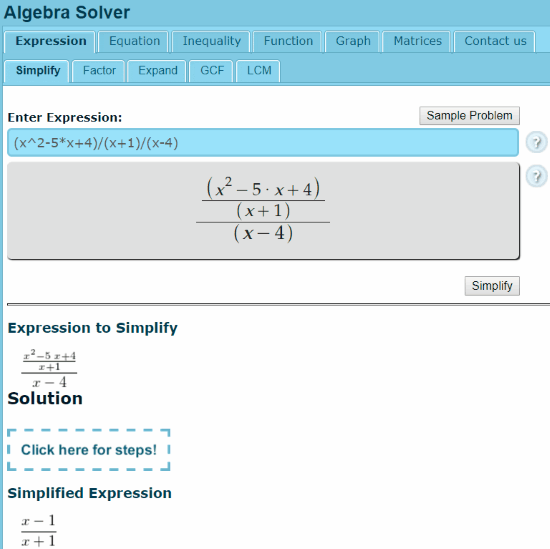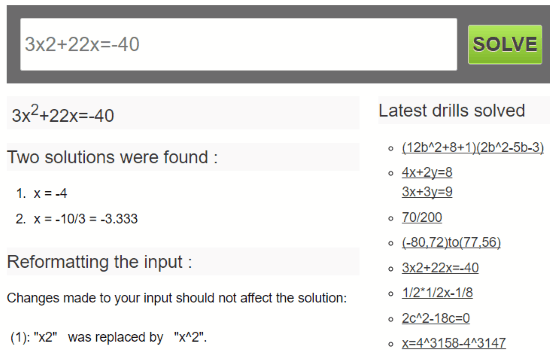Editor Ratings:
User Ratings:
[Total: 2 Average: 3]

In this article, I have compiled a list of 7 free websites to calculate algebra online. So, next time you are stuck on any maths problem, particularly pertaining to algebra, you can use any of these websites as your maths tutor to get their step by step solution. It doesn’t matter how complex or simple the algebraic expressions are; the algebra calculators on my list can solve math problems in just a few seconds. Well, some of them can even be used to solve trigonometric, exponential and logarithmic expressions and yes, of course, get their step by step solution. In some of them, you can also solve quadratic expressions and find their roots or solution, evaluate an expression by specifying values of variables, and so on. So, I have got covered all the algebra stuff for you in this article. Before getting started, grab all your algebra problems and be ready to solve them with these free online algebra calculators. In case you are looking for some apps, then check out these equation solver Android apps.

## Mathpapa:Mathpapa is the first free website on my list to calculate algebra online. With its advanced algebra calculator, you can solve linear equations, quadratic equations, simple mathematical expressions, etc. The best part is that it can also show you the step by step solution to the problem, as shown in the screenshot above. You don’t need to create any account to use its step by step algebra calculator; just visit the link provided above and specify the equation in the large text box and then hit the “CALCULATE IT!” button. It then solves the algebraic equations and displays its step by step solution. What I liked the most about its algebra calculator is that it has also got an “Evaluate” feature. In this feature, you can specify values of variables that you simplified first to get the solution. Again, it shows a step by step solution for this too. You must definitely take a look at it.

## Cymath:Cymath is another interesting website to calculate algebra step by step online. What I really liked about this free maths solver website is the fact that it also explains the concepts or formulae being used in a particular step. That is pretty awesome as kids can totally understand what’s going on while going through the steps which ultimately help them to figure out how to solve similar algebraic problems easily. You can solve different type of algebra problems like simplify, solve, and factor expressions, complete the square, plot a function, etc. Not just algebra, it can even solve integration and differentiation related problems step by step. Visit the link provided above and then simply enter your algebraic expression and click on the “Solve!” button. Cymath will then solve the expression and display a step by step solution, as shown in the screenshot above. Also, you can select a particular math topic like integration, differentiation, etc. before solving an expression to solve. Do check it out, you will thank me later :)

## QuickMath:QuickMath is another free online step by step algebra solver. With this website, you can solve a number of different algebra problems like solve equations, plot graphs for equations, find factors, simplify expressions, reduce fractions, partial fractions, plot graph for inequalities, etc. Similar to the previous website to calculate algebra online, this one also provides step by step solutions, as you can see in the screenshot above. This online algebra solver requires no login and signup; simply go to the link provided above and then enter your expression. After that, click on the “Simplify” button and your algebraic expression will be solved and a step by step solution will be presented to you. Do note that it also displays the specified expression as you would jot it down in your notebook.

## Symbolab:Symbolab is quite an advanced math solver website which can undoubtedly solve all your algebra-related problems. However, in its free version, you will not get step by step solution to your problems but it does give some steps as tricks and even explain the concept to be used to solve the algebraic question. In addition to algebra, you can also use it to solve other mathematical problems like integration, differentiation, arithmetic and geometric sequences, etc. As you can see in the screenshot above, I used it to solve a quadratic expression and how it explained the each step of the solution. It even mentioned the formula required to solve quadratic expressions. As you can see how well it can solve your problems, you should definitely check it out from the link above. All you have to do is specify an expression and then it will solve it and present its answer and some important steps in front of you.

You can use it to solve following algebra problems:

• Equations:
• Polynomial
• Inequalities:
• Linear
• Basic Operations:
• Simplify
• Factor
• Expand
• Join, etc.
• Algebraic Properties:
• Exponents
• Logarithms
• Absolute Value, etc.

## Mathway:Mathway is an online bot to solve algebra online. You can put this bot to solve and simplify algebraic expressions, find roots, perfect square, GCF, vertex form, domain and range, etc. The main limitation is that in its free version you won’t get any steps in the solution. However, I will still recommend it in case you just opt to look for answers to your problems and not the complete solutions. Go to the link provided above and then use its on-screen keyboard to type in the algebraic expressions. Once you have typed the expression, hit the Enter button to send the expression to this bot. It then prompts you to select an operation that you want to perform on the expression i.e. find roots, domain and range, simplify, etc. Once you select an operation, it instantly replies with the answer to your problem, as shown in the screenshot above.

## HomeschoolMath:HomeschoolMath is a free website to learn maths online. It also offers a free Algebra calculator which you can use to solve most of your maths problem related to algebra, like, simplify expressions, solve equations, calculator factors, solve matrix related problems, etc. However, it also doesn’t provide a step by step solution to your problems in its free version. If you wish to see a step by step solution, then I will recommend you to check out other tools to calculate algebra online from this list. Visit the link provided above and then specify the algebraic expression to solve. After that, click on the “Simplify” button to get the solution, as shown in the screenshot above.

## TigerAlgebra:TigerAlgebra is another simple website on my list to calculate algebra online. From it, you solve and simplify linear, quadratic, and exponential expressions. Similar to quite a few online algebra calculators on my list, it also doesn’t provide any step by step solution. Visit the link provided above and then enter the algebraic expression in the large text box, as shown in the screenshot above. After that, as soon as you click on the “SOLVE” button, it will return the answer of the expression.

### Verdict:

Next time you are stuck on any algebra related problems, then these websites will help you solve them in a flash. From these 7 free websites to calculate algebra online, I will recommend you to check out MathPapa, Cymath, and QuickMath as they provide a step by step solutions to your math problems.

 Editor Ratings: User Ratings:[Total: 2 Average: 3]